Basics of Geometry
edHelper subscribers - Create a new printable

Number of Keys
 Select the number of different printables: 1 key 2 keys 3 keys 4 keys 5 keys

Number of Pages (for each key)
 Select the number of pages: 1 page 2 pages 3 pages 4 pages

Include an answer key (answer keys will be at the end of the printable)
High School Geometry
High School Geometry
Math

 Name _____________________________Date ___________________ (Key 1 - Answer ID # 0734917)
1.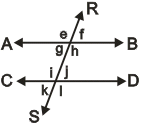AB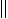CD

Name all of the angles that must be congruent to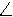k.
2.ABCD

mf = (65 + x)mk = (137 + 4x)Find the value of x.
3.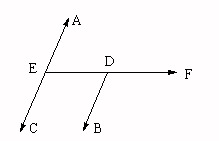Given:DBAC

mAED = (2x + 24)mBDF = (4x + 72)mEDB = (70 + 3y)Find the value of x and y.
4.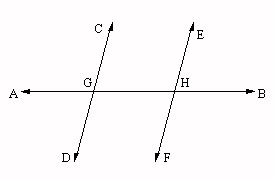CDEF

mDGH = (2x - 22)mFHG = (x + 133)Find the value of x.
5.   A transversal cuts two parallel lines.P andS are two interior angles on the same side of the transversal.
Given:
mP = (2x + 151)mS = (179 + x)What is the value of x?
6.   A transversal cuts two parallel lines.P andN are two alternate interior angles.
Given:
mP = (2x - 20)mN = (4 + x)What is the value of x?

Basics of Geometry
edHelper subscribers - Create a new printable

Number of Keys
 Select the number of different printables: 1 key 2 keys 3 keys 4 keys 5 keys

Number of Pages (for each key)
 Select the number of pages: 1 page 2 pages 3 pages 4 pages